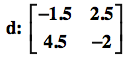### Home > A2C > Chapter Ch9 > Lesson 9.2.2 > Problem9-97

9-97.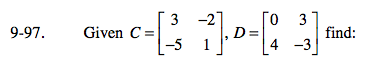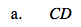Multiply each row of the first matrix into each column of the second matrix.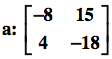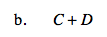Add the numbers in the corresponding entries.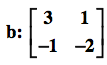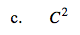C 2 = C · C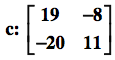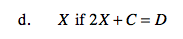2X + C = D
2X = D − C

$\textit{X}= \frac{(\textit{D}-\textit{C})}{2}$